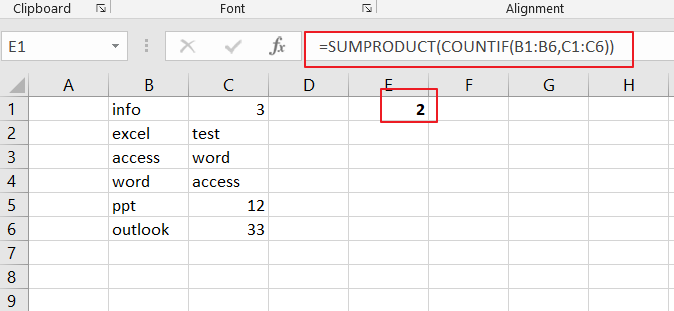# How to Count Total Matches in Two Ranges in Excel

This post will guide you how to count total matches in two given ranges in Excel 2013/2016 or Excel office 365. How do I count compare two ranges and count total matches between two given ranges in Excel.And you can do this by a formula based on the SUMPRODUCT  function and the COUNTIF function in Excel.

General Formula:

The below general formula can help you to compare two ranges and count total matches between the two ranges in Excel. Like this:

=SUMPRODUCT(COUNTIF(range1,range2)

## Count Total Matches in Two Ranges

Assuming that you have two range of cells(B1:B6 and C1:C6) that contains some of the same values, and you want to get a formula to compare these two ranges to see how many of cells in range 2 appear in the range1. You also do not care about the order of that items in two ranges, and you just want to know how many values in range 2 that appear in range1.

=SUMPRODUCT(COUNTIF(B1:B6,C1:C6)You can use the COUNTIF function to count cells in a range that match your given condition. For example, you can use the COUNTIF function to count a range like B2:B6 and also give a simple criteria like “=45”. And the function would return the count of cells in range B2:B6 that are equals to numeric value 45.

If you only want to use the COUNTIF function to check for equivalency for a given range, you do not need to use any logic operators.

Because two ranges are as an argument in the COUNTIF function, and each of range that contain 6 cell values, COUNTIF function will return an array result that contain 6 items. And each value in the array result that represents a count. That array result looks like this:

Then you still need to add up the items in the above array. And you can use the SUMPRODUCT function to sum up the items in that array list. If there are two or more array lists, and the SUMPRODUCT will multiple the arrays together, and sump up the results.

### Related Functions

• Excel SUMPRODUCT function
The Excel SUMPRODUCT function multiplies corresponding components in the given one or more arrays or ranges, and returns the sum of those products.The syntax of the SUMPRODUCT function is as below:= SUMPRODUCT (array1,[array2],…)…
• Excel COUNTIF function
The Excel COUNTIF function will count the number of cells in a range that meet a given criteria. This function can be used to count the different kinds of cells with number, date, text values, blank, non-blanks, or containing specific characters.etc.= COUNTIF (range, criteria)…